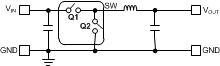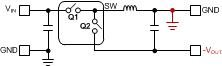# LM5017 Based Inverting Buck-Boost Enables Negative Supply

Other Parts Discussed in Post: LM5017

The synchronous buck converter IC can be used in inverting buck-boost configuration by simple modifications to buck converter schematic as shown in Figure 1a and 1b. The inverting buck-boost converter generates output voltage of negative polarity given by: VOUT= -D/(1-D) x VIN1a. A synchronous buck converter 1b: Inverting buck-boost converter

Figure 1. Using a buck regulator IC as an inverting buck-boost converter

The operation of the inverting buck-boost converter is shown in Figure 2a and 2b. During TON (Q1:ON, Q2:OFF) the inductor stores energy and during TOFF (Q1:OFF, Q2:ON) the inductor charges the output capacitor.

Figure 2. Inverting buck-boost operation

Maximum VIN and IOUT of a Buck IC in Inverting Buck-Boost Configuration

When using a buck regulator IC in inverting configuration the maximum input voltage and the maximum output current range of the converter needs to be reduced. To illustrate these concepts, an LM5017 based inverting buck-boost circuit is shown in Figure 3. In this configuration, the bias ground of the IC (RTN pin) is connected to the negative output voltage (-10V). The voltage across the input (VIN) and return (RTN) terminals of IC is given by:
VIN, RTN = VIN + |VOUT|

The maximum input voltage is therefore given by: VIN(MAX) = VIN,RTN (MAX) - |VOUT|

As the inductor current supplies the output capacitor only during TOFF (Figure 2b), the output current is given by: IOUT = IL1 (1-D)

where D is the duty cycle. The maximum output current is related to the switch current limit by the following equation:
iL1(peak) = iSW(peak) = IL1+ΔIL1/2 = IOUT/(1-D) + ΔIL1/2

where ΔIL1 is the peak to peak inductor current ripple which peaks at the maximum VIN:

A complete LM5017 based inverting buck-boost converter is shown in Figure 3. Because of the wide VIN (100V) rating of LM5017 it can operate from wide input voltage rails even in the inverting buck-boost application.

Figure 3. A 10 V - 60 V input to -10 V output, 300 mA inverting buck-boost application circuit